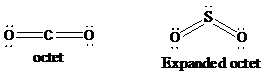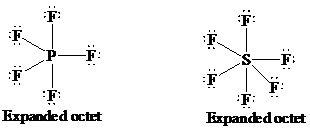# The molecules which does not obey octet rule are

1. A
${\text{PF}}_{5}$
2. B
${\text{SF}}_{6}$
3. C
${\text{SO}}_{2}$
4. D
${\text{CO}}_{2}$

FREE Lve Classes, PDFs, Solved Questions, PYQ's, Mock Tests, Practice Tests, and Test Series!

+91

Verify OTP Code (required)

I agree to the terms and conditions and privacy policy.

### Solution:

In a molecule,  the sum of valence electrons of  all atoms is equal to

8 then it is said to be an octet. The molecules which obey octet rule

they have high stability.## Related contentJoin Infinity Learn Regular Class Program!

Sign up & Get instant access to FREE PDF's, solved questions, Previous Year Papers, Quizzes and Puzzles!

+91

Verify OTP Code (required)

I agree to the terms and conditions and privacy policy.